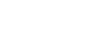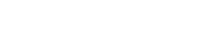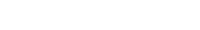# Random Variable: What is it in Statistics?

Types of Variable > Random Variable

Contents:

## What is a Random Variable?

In algebra you probably remember using variables like “x” or “y” which represent an unknown quantity like y = x + 1. You solve for the value of x, and x therefore represents a particular number (or set of numbers, if you’re talking about a function). Then you get to statistics and different kinds of variables are used, including random variables. These variables are still quantities, but unlike “x” or “y” (which are simply just numbers), random variables have distinct characteristics and behaviors:

1. Random variables are denoted by capital letters. If you see a lowercase x or y, that’s the kind of variable you’re used to in algebra. It refers to an unknown quantity or quantities. If you see an uppercase X or Y, that’s a random variable and it usually refers to the probability of getting a certain outcome.
2. Random variables are associated with random processes.A random process is an event or experiment that has a random outcome. For example: rolling a die, choosing a card, choosing a bingo ball, playing slot machines or any one of hundreds of thousands of other possibilities. It’s something you can’t exactly predict an outcome for; you might have a range of possibilities so you calculate the probability of a particular outcome.
3. Random variables give numbers to outcomes of random events. Random variables are numerical in the same way that x or y is numerical, except it is attached to a random event.

## Discrete and Continuous Random Variables

Random variables can be discrete or continuous.

Discrete random variables have the following properties :

Continuous random variables share similar properties:

## Random Event Examples

Rolling a die is a random event and you can quantify (i.e. give a number to) the outcome. Let’s say you wanted to know how many sixes you get if you roll the die a certain number of times. Your random variable, X could be equal to 1 if you get a six and 0 if you get any other number.
This is just an example; You can define X and Y however you like (i.e. 2 if you roll a six and 9 if you don’t).

A few more example of random variables:

• X = total of lotto numbers.
• Y = number of open parking spaces in a parking lot.
• Z = number of aces in a card hand.

## Random Event Probabilities

Random variables are most often used in conjunction with a probability of a random event happening. Say you wanted to see if the probability of getting four aces in a hand when playing cards is less than 5 percent. You could write it as:
P (getting four aces in a hand of 52 cards when four are dealt at a time <.05) = That can get kind of wordy, especially if you have to write it over and over. If you define the random variable, X getting four aces in a hand: X = getting four aces in a hand of 52 cards when four are dealt at a time then you can write: P (X < .05) because you've defined X. If you are familiar with computer programming, it's a very similar concept to defining variables in a programming language so that your later calculations can draw on those variables. The good news is that in elementary statistics or AP statistics, the random variables are usually defined for you, so you don’t have to worry about defining them yourself.

In calculus based statistics, the probability of a random variable can be defined as a definite integral :
The probability that a ≤ X ≤ b is:Where fX is the pdf of X.

## Mean and mode of a Random Variable

The mean of a discrete random variable is the weighted mean of the values. The formula is:
μx = x1*p1 + x2*p2 + hellip; + x2*p2 = Σ xipi.
In other words, multiply each given value by the probability of getting that value, then add everything up.

For continuous random variables, there isn’t a simple formula to find the mean. You’ll want to look up the formula for the probability distribution your variables fall into. For example, the mean for the normal distribution is the center of the curve, while the mean for the uniform distribution is b + a / 2.

The mode for continuous random variables with pdf fX can be found with optimization, by setting the derivative equal to zero. Specifically, a local maximum of fX where the first derivative of fX is zero and the second derivative is less than or equal to zero. In prime notation, that’s any point x with:
f′X(x) = 0 and f′′X(x) ≤ 0.

## Variance of a Random Variable

The formula for calculating the variance of a discrete random variable is:

σ2 = Σ(xi – μ)2f(x)

Note: This is also one of the AP Statistics formulas.

## Variance of a Random Variable: Steps

Example problem: Find the variance of X for the following set of probability distribution data which represents the number of misshapen pizzas for every 100 pizzas produced in a certain factory:

• x: 2, 3, 4, 5, 6
• f(x): 0.01, 0.25, 0.4, 0.3, 0.4.

Step 1: Multiply each value of x by f(x) and add them up to find the mean, μ:

• 2 * 0.1 +
• 3 * 0.25 +
• 4 * 0.4 +
• 5 * 0.3 +
• 6 * 0.4 =
• 4.11

Step 2: Use the variance formula to find the variance. This time we’re going to subtract the mean, μ, from each x-value, square it, and then multiply by the f(x) values:
σ2 = Σ(xi-μ)2f(p) =

• (2 – 4.11)2(0.01) +
• (3 – 4.11)2(0.25) +
• (4 – 4.11)2(0.4) +
• (5 – 4.11)2(0.3) +
• (6 – 4.11)2(0.04) =
• 0.74

The variance of the random variable is 0.74
That’s it!

Example 2: Variance of a Discrete Random Variable (Probability Table)
Question: Find the variance for the following data, giving the probability (p) of a certain percent increase in stocks 1, 2, and 3:Step 1: Find the expected value (which equals the mean of the distribution):
=((-4.00% * 0.22) + (5.00% * 0.43) + (16.00%*0.35)) = 6.87%.

Step 2: Subtract the mean from each X-value, then square the results:

• (-4.00% – 6.87%)2 = 118.1569
• (5.00% – 6.87%)2 = 3.4969
• (16.00% – 6.87%)2 = 83.3569

Step 3: Multiply the results in Step 2 by their associated probabilities (from the table):

• 118.1569 * 0.22 = 25.9945
• 3.4969 * 0.43 = 1.5037
• 83.3569 * 0.35 = 29.1749

Step 4: Add the results from Step 3 together:

• 25.9945 + 1.5037 + 29.1749= 56.67%

## Variance of a Continuous Random Variable

It is possible to calculate the variance of a continuous random variable using calculus.

## Binomial Random Variable

A binomial random variable is a count of the number of successes in a binomial experiment.Rolling dice can be a binomial experiment under the right conditions.
For a variable to be classified as a binomial random variable, the following conditions must all be true:

• There must be a fixed sample size (a certain number of trials).
• For each trial, the success must either happen or it must not.
• The probability for each event must be exactly the same.
• Each trial must be an independent event.

## Examples of binomial random variable

• The number of heads when you flip a fair coin 30 times.
• Number of winning scratch-off lottery tickets when you purchase 20 of the same type.
• Number of people who are right-handed in a random sample of 200 people.
• Number of people who respond “yes” to whether they voted for Obama in the 2012 election.
• Number of Starbucks customers in a sample of 40 who prefer house coffee to Frappuccinos.

Two important characteristics of a binomial distribution (random binomial variables have a binomial distribution):

1. n = a fixed number of trials.
2. p = probability of success for each trial.

For example, tossing a coin ten times to see how many heads you flip: n = 10, p = .5 (because you have a 50% chance of flipping a head).

Tips:

1. If you aren’t counting something, then it isn’t a binomial random variable.
2. The number of trials in your experiment must be fixed. For example, “the number of times you roll a die before rolling a 3” is not a binomial random variable, because there is an indefinite number of trials. On the other hand, rolling a die 30 times and counting how many times you roll a 3 is a binomial random variable.

## Probability Distribution and CDF

The probability distribution function (PDF) for a continuous random variable can be described by the integral :The PDF f(x) satisfies the following two properties:

The PDF doesn’t tell us what the probabilities are though (e.g. P(X < 5) or P(X = 6). For that, we need a different formula. For continuous random variables, the probability of an event X can be calculated with the integral :Where f(x) is the PDF.

As this is an integral, it makes sense that the probability of any one particular outcome is zero. Another way to think of this: if you measure the length of a car with infinite precision, the probability of another car having exactly the same length is zero.

The CDF is the integral:Example:
The following video shows how to find the cumulative distribution function for a random variable with pdf f(x) = 3x2, 0 < 1:

Watch this video on YouTube.

## References

 Orloff, J. & Bloom, J. Continuous Random Variables. Retrieved April 29, 2021 from: https://ocw.mit.edu/courses/mathematics/18-05-introduction-to-probability-and-statistics-spring-2014/readings/MIT18_05S14_Reading5b.pdf
 Kjos-Hanssen, B. Statistics for Calculus Students. Retrieved April 29, 2021 from: https://dspace.lib.hawaii.edu/bitstream/10790/4572/s4cs.pdf

CITE THIS AS:
Stephanie Glen. "Random Variable: What is it in Statistics?" From StatisticsHowTo.com: Elementary Statistics for the rest of us! https://www.statisticshowto.com/random-variable/
---------------------------------------------------------------------------Need help with a homework or test question? With Chegg Study, you can get step-by-step solutions to your questions from an expert in the field. Your first 30 minutes with a Chegg tutor is free!